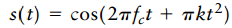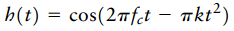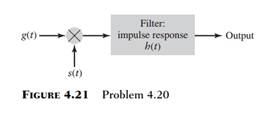Create an Account

Home / Questions / Figure 4 21 shows the block diagram of a real time spectrum analyzer working on the princi...

Figure 4 21 shows the block diagram of a real time spectrum analyzer working on the principle of frequency modulation The given signal gtand a frequency modulated signal stare applied to a

Figure 4.21 shows the block diagram of a real-time spectrum analyzer working on the principle of frequency modulation. The given signal g(t)and a frequency-modulated signal s(t)are applied to a multiplier and the output g(t) s(t) is fed into a filter of impulse b(t).The and s(t) are linear FM signals whose instantaneous frequencies vary at opposite rates, as shown byAndwhere k is a constant. Show that the envelope of the filter output is proportional to the amplitude spectrum of the input signal g(t) with the product term kt playing the role of frequency f.Jul 31 2020 View more View LessSubscribe To Get Solution